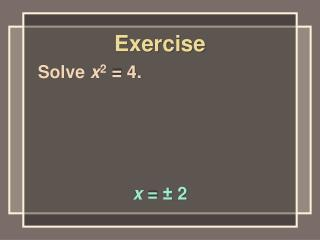Download PresentationExercise

# Exercise - PowerPoint PPT PresentationDownload Presentation## Exercise

- - - - - - - - - - - - - - - - - - - - - - - - - - - E N D - - - - - - - - - - - - - - - - - - - - - - - - - - -
##### Presentation Transcript

1. Exercise Solve x2 = 4. x = ± 2

2. Exercise Solve x2 = –4. no real solution

3. Exercise Solve √x = 4. x = 16

4. Exercise Solve √x = –4. no real solution

5. Exercise Solve √–x = 4. x = –16

6. hypotenuse leg leg

7. 25 square units 9 square units 3 5 4 16 square units

8. 3 5 4 25 square units 9 square units += 16 square units

9. 42 32 52 += 16 9 25

10. The Pythagorean Theorem If the hypotenuse of a right triangle has length c, and the legs have lengths a and b, then a2 + b2 = c2.

11. √c2 = √289 Example 1 Find the hypotenuse of a right triangle with legs of 8 and 15. c2 = a2 + b2 c2 = 289 c2 = 82 + 152 c2 = 64 + 225 c = 17

12. √c2 = √85 c = √85 ≈ 9.2 Example 2 Find the hypotenuse of a right triangle with legs of 6 and 7. c2 = a2 + b2 c2 = 85 c2 = 62 + 72 c2 = 36 + 49

13. Example Find the hypotenuse of a right triangle with legs of 9 and 12. 15

14. Example Find the hypotenuse of a right triangle with legs of and . 12 √32 1

15. √2 Example Find the hypotenuse of a right triangle with legs of 1 and 1.

16. a = √207 ≈ 14.4 Example 3 Find the leg of a right triangle whose hypotenuse is 16 and other leg is 7. a2 + 72 = 162 a2 + 49 = 256 a2 + 49 – 49 = 256 – 49 a2 = 207

17. Example Find the length of a leg of a right triangle whose hypotenuse is 39 and whose other leg is 15. 36

18. √300 ≈ 17.3 Example Find the length of a leg of a right triangle whose hypotenuse is 20 and whose other leg is 10.

19. Converse The converse is the statement resulting when the “if” part and the “then” part of a conditional statement are switched.

20. Converse of the Pythagorean Theorem If a triangle has sides a, b, and c, such that a2 + b2 = c2, then the triangle is a right triangle.

21. Example 4 Determine whether a triangle with sides of 12, 35, and 37 is a right triangle. a2 + b2 = c2 yes 122 + 352 = 372 144 + 1,225 = 1,369 1,369 = 1,369

22. Example 5 Determine whether a triangle with sides of 8, 12, and 14 is a right triangle. a2 + b2 = c2 no 82 + 122 = 142 64 + 144 = 196 208 ≠ 196

23. Example Determine whether a triangle with sides of 15, 18, and 22 is a right triangle. no; 152 + 182 ≠ 222

24. Example Determine whether a triangle with sides of 16, 30, and 34 is a right triangle. yes; 162 + 302 = 1,156 = 342

25. Exercise A 16 ft. ladder leans up against the side of a building. If the base of the ladder is 4 ft. from the base of the building, how high up the side of the building will the ladder reach? 15.5 ft.

26. Exercise A 200 ft. tower is braced to the ground by a cable, from a point 150 ft. above the ground to a point 87 ft. from the base of the tower. How long is the cable? 173.4 ft.

27. Exercise The distance between bases on a baseball diamond is 90 ft. How far is it from home plate to second base? 127.3 ft.

28. Exercise An opening for a window is 23” wide, 54” tall, and 60” diagonally. Is the opening “square”; that is, do the height and width form a right angle? no